# The Backprop Algorithm¶

In :
from ipypublish import nb_setup


## Vast Scale of Gradient Descent in DLNs¶

In Chapter LinearLearningModels we formulated the parameter estimation (or learning) problem for Linear Systems and showed that it could be solved using an optimization procedure based on the Gradient Descent algorithm. We now extend this framework to Dense Feed Forward Networks. Indeed all the arguments in Chapter LinearLearningModels for Linear Systems continue to hold for Dense Feed Forward Networks, the only difference being that we can no longer use the simple gradient calculation

$$\frac{\partial \mathcal L}{\partial w_{ki}} = x_i(y_k - t_k)$$

The gradients are instead computed by an algorithm called Backprop, which is probably the single most important algorithm in all of Deep Learning. Backprop was discovered in the mid-1980s, and since then the base algorithm hasn't changed and it is still the most efficient technique that we know of to compute gradients in DLN systems.

In order to give an idea of the magnitude of the problem, consider a multi-layer Dense Learning model of the type discussed in Chapter NNDeepLearning. Models of this type can have millions of parameters: For example consider the case when the input is a 224x224x3 color image, and the model has a single Hidden Layer with 100 nodes. The number of weights in just the first layer of connections between the Input and the Hidden Layer is given by 224x224x3x100 = 15,082,800! In order to estimate these weights from the training dataset, the gradients $\frac{\partial\mathcal L}{\partial w_{ij}}$ with respect each one of these weights need to be calculated. This is a fairly daunting task, and a lack of solution stymied progress in DLN system until the mid-1980s. The logjam was finally broken with the discovery of the Backprop algorithm, see for example Montavon, Orr, and Müller (2012). Our objective is to derive this algorithm as well provide some intuition into the subject of Gradient Flows in general. Most of the advances in DLN Architecture in the last few years have been made with the objective of improving Gradient Flows within the network.

## Optimization Problem¶

We start by stating the optimization problem for Dense Feed Forward Networks. The system parameters are estimated by solving the following optimization problem: Find the model parameters that minimize the Loss Function $L$ given by:

where the Cross Entropy Function $\mathcal L$ for a single training sample is given by

$$\mathcal L = -\sum_{k=1}^K t_k\log y_k$$

In this equation $(t_1,...,t_K)$ is the Label or Ground Truth vector and $(y_1,...,y_K)$ is the vector of classification probabilities, given by

$$y_k = \frac{\exp(a_k)}{\sum_{j=1}^K\exp(a_j)},\ \ 1\le k\le K$$

The numbers $(a_1,...,a_K)$ are the logits and are computed using a scoring function $h_k$, so that

$$a_k = h_k(W, b),\ \ 1\le k\le K$$

The numbers $(W,b)$ represent the parameters that are used to specify the model. For Linear Systems the scoring functions were fairly simple:

$$a_k = \sum_{i=1}^N w_{ki}x_i + b_k,\ \ 1\le k\le K$$

For Dense Feed Forward Networks, these functions are much more complex. For example for a network with a single Hidden Layer the equations are

$$a_k = \sum_{i=1}^P w_{ki}^{(2)}z_i + b_k^{(2)},\ \ 1\le k\le K\,\ \ \mbox{where}\ \ z_i = f(\sum_{j=1}^N w_{ij}^{(1)}x_j + b_{i}^{(1)}),\ \ 1\le i\le P$$

where $f$ is the Activation Function.

This optimization problem is solved by using the Gradient Descent Algorithm, according to which the optimal parameters $(W,b)$ are estimated iteratively using the following equations:

$$w_{ij} \leftarrow w_{ij} - \frac{\eta}{M}\sum_{m=1}^M \frac{\partial\mathcal L(m)}{\partial w_{ij}}$$$$b_i \leftarrow b_i - \frac{\eta}{M}\sum_{m=1}^M \frac{\partial{\mathcal L}(m)}{\partial b_i}$$

As was discussed in Chapter LinearLearningModels, there are two other Optimization Algorithms that are used in practice, since they converge faster than Gradient Descent, namely Stochastic Gradient Descent (SGD), given by

$$w_{ij} \leftarrow w_{ij} - \eta \frac{\partial{\mathcal L}(m)}{\partial w_{ij}}$$$$b_i \leftarrow b_i - \eta \frac{\partial{\mathcal L}(m)}{\partial b_i}$$

and Batch Stochastic Gradient Descent (B-SGD) with batch size $B$, given by:

$$w_{ij} \leftarrow w_{ij} - \frac{\eta}{B}\sum_{m=1}^{B} \frac{\partial{\mathcal L}(m)}{\partial w_{ij}}$$$$b_i \leftarrow b_i - \frac{\eta}{B}\sum_{m=1}^{B} \frac{\partial{\mathcal L}(m)}{\partial b_i}$$

In the absence of an explicit formula for $\frac{\partial{\mathcal L}}{\partial w_{ij}}$, how do we proceed forward? A brute force approach to estimating these gradients is the following:

Do a couple of forward passes through the network to compute $\mathcal L(w_{11},...,w_{ij},..,w_{MN})$ and $\mathcal L(w_{11},...,w_{ij} + \epsilon,..,w_{MN})$, where $\epsilon$ is a small increment. Using these numbers the gradient with respect to $w_{ij}$ can be estimated

$$\frac{\partial {\mathcal L}}{\partial w_{ij}} = \frac{ {\mathcal L}(w_{11},...,w_{ij} + \epsilon,..,w_{MN}) - {\mathcal L}(w_{11},...,w_{ij},..,w_{MN})}{\epsilon}$$

The reader can see that there is a problem with this scheme: If the network has a million parameters, then this requires a million and one forward passes through the network! The Backprop algorithm on the other hand, only requires just two passes through the network, irrespective of the number of parameters! As Figure backprop1 shows, Backprop requires a forward pass during which all the activations in the network are computed, and this is followed by a backward pass to the compute the gradients.

In :
#backprop1
nb_setup.images_hconcat(["DL_images/backprop1.png"], width=600)

Out: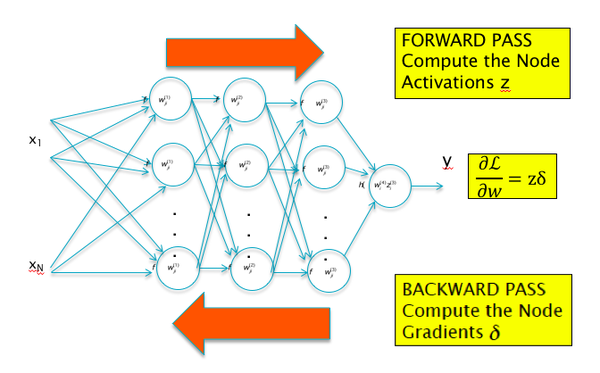## The Chain Rule of Derivatives¶

The fundamental difficult in computing $\frac{\partial {\mathcal L}}{\partial w_{ij}}$ arises from the following: The function $\mathcal L$ can written as a nested composition of multiple sub-functions $f, g, h$ etc, such that the weight $w_{ij}$ is buried several layers deep into it, i.e. $\mathcal L$ can be written as $\mathcal L = f(w_{.}..g(w_{.}..h(w_{ij}..))$. In the case of Neural Networks, each of these functions $f, g, h$ by themselves are fairly simple, such as additions, max or multiplication by a constant, but after composition, the resulting function $f(g(h(...)))$ assumes a very complex form, which makes computation of $\frac{\partial {\mathcal L}}{\partial w_{ij}}$ by direct differentiation difficult.

In :
#BP1
nb_setup.images_hconcat(["DL_images/BP1.png"], width=600)

Out: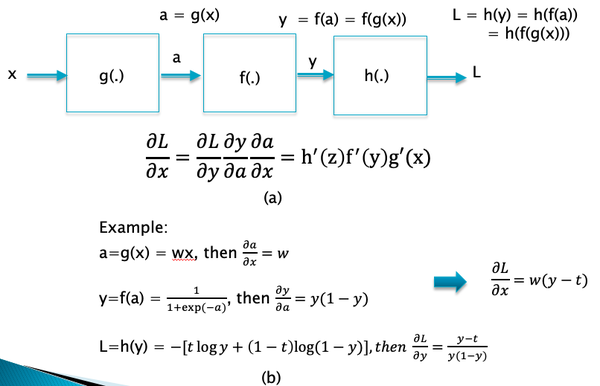Fortunately there is a result from Differential Calculus called the Chain Rule of Derivatives which can be used to simplify this computation. Indeed the Backprop algorithm can be considered to be an application of this rule.

In order to understand the Chain Rule, consider Part (a) of Figure BP1, which shows the computation of $L$ as a composition of the functions $g$, $f$ and $h$ in series. The Chain Rule says that the end-to-end dervative ${{\partial L}\over{\partial x}}$ can be expressed as product of the derivatives across each one of the functions, i.e.

$${{\partial L}\over{\partial x}} = {{\partial L}\over{\partial y}}{{\partial y}\over{\partial a}}{{\partial a}\over{\partial x}}$$

An example of the application of this rule is shown on Part (b) of Figure BP1, which we actually encountered in the chapter on Linear Models.

There is an important extension to the Chain Rule which is also used extensively in Backprop. As shown in backprop3, this arises for the case in which the output $L$ is a function of multiple inputs $(y_1,...,y_K)$, and each of these are in turn functions of their own inputs $(a_1,...,a_N)$.

In :
#backprop3
nb_setup.images_hconcat(["DL_images/backprop3.png"], width=600)

Out: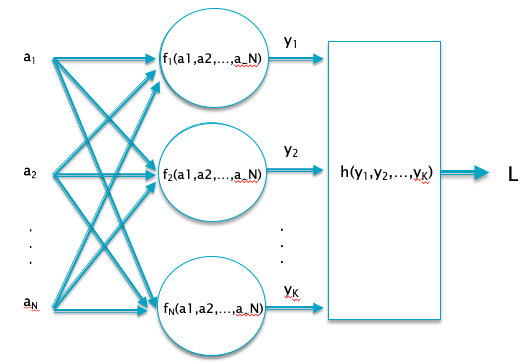In this case, the Chain Rule of Differentiation leads to the following rule:

$$\frac{\partial{\mathcal L}}{\partial a_i} = \sum_{k=1}^K \frac{\partial{\mathcal L}}{\partial y_k} \frac{\partial{y_k}}{\partial a_i}$$

An important application of this rule is at the output of the Dense Feed Forward Network:

$$\mathcal L = -\sum_{j=1}^K t_j\log y_j,\ \ \mbox{and}\ \ y_j = \frac{\exp(a_j)}{\sum_{k=1}^K \exp(a_k)},\ \ 1\le j\le K$$

Since these equations are also applicable to the output of Linear Models, the computation of $\frac{\partial{\mathcal L}}{\partial a_i}$ was actually carried in Chapter LinearLearningModels where we showed that (see Section Multiclass Logistic Regression in that chapter)

$$\frac{\partial{\mathcal L}}{\partial a_i} = y_i - t_i,\ \ 1\le i\le K$$

In :
#backprop2
nb_setup.images_hconcat(["DL_images/backprop2.png"], width=600)

Out: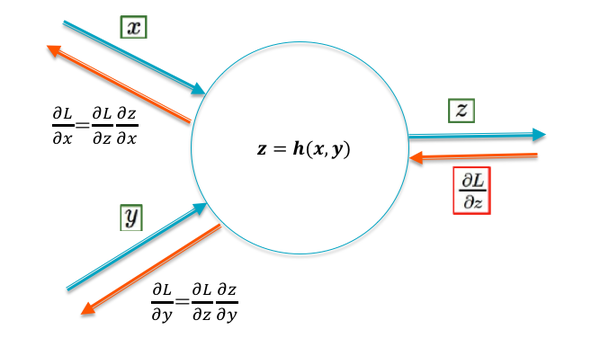Gradient Flow Calculus is the set of rules used by the Backprop algorithm to compute gradients (this also accounts for the use of the term "flow" in tools such as Tensor Flow). Backprop works by first computing the gradients $\frac{\partial{\mathcal L}}{\partial y_{k}}, i\le k\le K$ at the output of the network (which can be computed using an explicit formula), then propagating or flowing these gradients back into the network. In this section we derive the rules that govern this backward flow of gradients.

Figure backprop2 shows a node with inputs $(x,y)$ and output $z = h(x,y)$, where $h$ represents the function performed at the node. Lets assume that the gradient $\frac{\partial{\mathcal L}}{\partial z}$ is known. Using the Chain Rule of Differentiation, the gradients $\frac{\partial{\mathcal L}}{\partial x}$ and $\frac{\partial{\mathcal L}}{\partial y}$ can be computed as:

$$\frac{\partial{\mathcal L}}{\partial x} = \frac{\partial{\mathcal L}}{\partial z}\frac{\partial{z}}{\partial x}$$$$\frac{\partial{\mathcal L}}{\partial y} = \frac{\partial{\mathcal L}}{\partial z}\frac{\partial{z}}{\partial y}$$
In :
#BP4
nb_setup.images_hconcat(["DL_images/BP4.png"], width=600)

Out: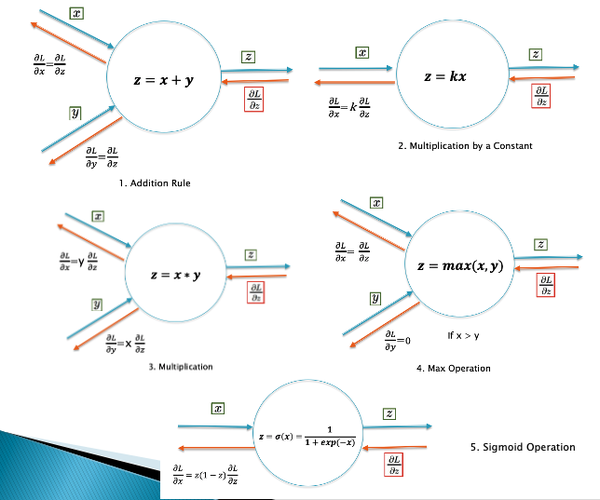This rule can be used to compute the effect of the node on the gradients flowing back from right to left. We apply these equations to the following cases (also shown in Figure BP4):

$$z = x+y$$

In this case

$$\frac{\partial{\mathcal L}}{\partial x} = \frac{\partial{\mathcal L}}{\partial y} = \frac{\partial{\mathcal L}}{\partial z}$$

Hence the addition operation broadcasts the incoming gradient $\frac{\partial{\mathcal L}}{\partial z}$ backwards to all its input branches, without any modification. This is a simple result, but quite important in DLN architecture.

2. Rule 2: Multiplication by a Constant

$$z = kx$$

which results in

$$\frac{\partial{\mathcal L}}{\partial x} = k\frac{\partial{\mathcal L}}{\partial z}$$

i.e., the multiplication operation in the forward direction results in an identical multiplication of the gradients flowing back.

3. Rule 3: Multiplication

$$z = xy$$

In this case

$$\frac{\partial{\mathcal L}}{\partial x} = y\frac{\partial{\mathcal L}}{\partial z}, \ \ \mbox{and}\ \ \frac{\partial{\mathcal L}}{\partial y} = x\frac{\partial{\mathcal L}}{\partial z}$$

Hence the multiplication operation also passes on the incoming gradient, but after multiplying it with the value from the other input branch.

4. Rule 4: The Max Operation

$$z = \max(x, y)$$$$\frac{\partial{\mathcal L}}{\partial x} = \frac{\partial{\mathcal L}}{\partial z}\ \ if\ \ x > y\ \ \mbox{and}\ \ 0\ \ \mbox{otherwise}$$

This shows that the max operation acts like a switch, so that the incoming gradient is switched to the input branch that is larger, while the other branch gets a zero gradient.

5. Rule 5: The Sigmoid Function

$$z = \sigma(x) = \frac{1}{1+\exp(-x)}$$

In this case

$$\frac{\partial{\mathcal L}}{\partial x} = z(1-z)\frac{\partial{\mathcal L}}{\partial z}$$

This equation has some very interesting implications for gradient flows for the case when the sigmoid is used as the Activation Function in DLNs. It implies that if the input value $x$ is very large or very small, then the corresponding gradient $\frac{\partial{\mathcal L}}{\partial x}$ goes to zero (to see why, see Figure sigmoid in Chapter Linear Learning Models). We will see in the next chapter that this behavior held back progress in DLNs for almost two decades, even after the discovery of the Backprop algorithm.

In :
#backprop4
nb_setup.images_hconcat(["DL_images/backprop4.png"], width=600)

Out: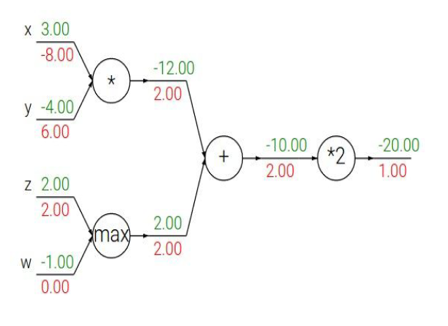Figure backprop4 illustrates an application of the Gradient Flow rules to a simple neural network. The numbers in green represent the forward flow of activations, while the red numbers represent the backward flow of gradients.

## Backprop¶

In :
#BP3
nb_setup.images_hconcat(["DL_images/BP3.png"], width=600)

Out: